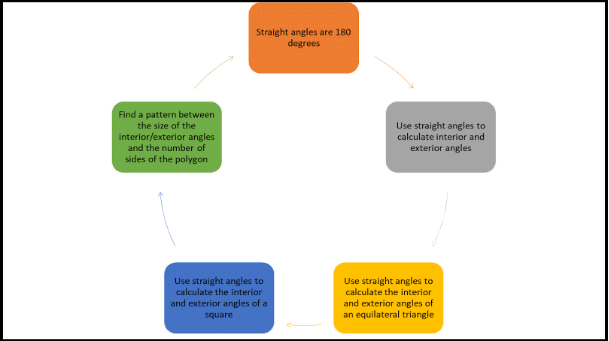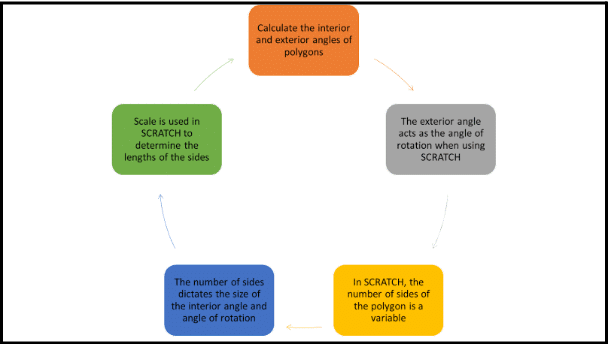# Shape and Space

CLASS

STRAND

#### Shape and Space

LEARNING OUTCOME LABEL

#### Goals of this activity

Children will:

1. Recognise the relationship between the number of sides of a polygon and the interior angles of the polygon. (Understanding and Connecting;  Reasoning).
2. Name and describe polygons using appropriate mathematical language. (Communicating).
3. Engage in problem solving to identify the relationship between the exterior angle of polygons and the interior angles. (Applying and  Problem Solving).
4. Identify angles of rotation in Scratch coding to create polygons. Explain reasoning in the identification of angles of rotation (Communicating, Reasoning).
1. A polygon is a 2D shape with straight sides. In a regular polygon, all sides are the same length and all angles are the same size.
2. We can use straight angles to calculate the exterior and interior angles of polygons. We can do this by using the interior angles of an equilateral triangle and interior angles of a square to develop a pattern and use this pattern to calculate the angles of multiple polygons.
3. We can use the knowledge of exterior angles to calculate the anti-clockwise rotations needed to create polygons using Scratch coding. The angle of rotation is calculated by identifying the exterior angle. The number of sides of the polygon needs to be identified and inputted into the code. The code also includes identifying the position of the polygon in relation to the axis. Scale is used when selecting the length of each side of the polygon.
 This table gives an overview of relevant content from the progression continua in the curriculum. The content most relevant to this lesson is shown in bold. Strand:  Measuring Learning Outcome Labels Shape Level E Level F Level I Level J Level K Understanding  and Connecting Explores movement from one location to another using a grid or simple map. Recognises and uses directions ‘right’ and ‘left’ in real situations.  Describes movement in terms of whole, half, quarter and three-quarter turns. Identifies and describes the general location of an object using a grid system.  Explores square and non-square corners in the environment, identifying square corners as right angles. Draws given angles and measures them in degrees.  Develops an understanding of the eight points of the compass and explore angles of 45°.  Explores the positions and types of angles [internal and external] in shapes.  Explores the sum of the angles in a triangle.  Explores, measures angles and constructs different types of triangles. Describes positions on the full coordinate grid (all four quadrants)  Explores the sum of the angles in quadrilaterals.  Explores, estimates and measures the angles formed when two lines intersect.  Explores and measures internal and external angles of crosssections of shapes. Describes and compares regular and irregular shapes defined in terms of co-ordinates. Communicating Gives and follows simple directions.  Uses language ‘clockwise, anticlockwise, right, left’ to describe turns and direction. Gives and follows directions involving half and quarter turns in the context of simple plans.  Describes and records directions as a series of simple steps.  Relate clockwise movements to the clockface.  Analyses and evaluates representations for accuracy Follows and gives turning instructions related to the eight points of the compass.  Represent coordinates in the first quadrant; including identifying position from given co-ordinates and plotting position. Draws a pair of axes [X and Y] to form a quadrant, considering equal scales and labelling. Represents coordinates in all four quadrants Reasoning Visualises the result of following a sequence of directions on a map or plan.  Deduces and identifies where, in a series of steps, the wrong direction may have been taken. Relates clockwise movements to the clockface. Recognises the relationship between different modes of representing position and location (For example – birds-eye view versus street view).  Evaluates directions for movement and refines for clarity and accuracy. Explores how a co-ordinate system represents location.  Constructs shapes given some properties and / or values. Uses angle sum facts to make deductions about missing angles.  Deduces the reasonableness of estimated measures of angles of intersecting lines. Explores angle relationships associated with parallel lines. Draw and labels rectangles [including squares], parallelograms and rhombuses, specified by coordinates in the four quadrants, predicting missing coordinates using the properties of shapes.

 Label E Label F Label I Label J Label K Applying and   Problem Solving Solves problems and plays games involving simple maps or grids. Creates a set of simple instructions to direct movement for a given purpose. Identifies and models turns using arms, legs, whole body movements or straws. Devises and analyses routes on maps, plans or grids that satisfy certain constraints (For example – the shortest route, no crossing roads, avoiding obstacles). Solves problems that involve missing angles.  Solves problems involving placing and locating coordinate points in the first quadrant Uses manipulatives or programmable devices to explore position, movement and direction.  Plots and connects coordinates to make an image. Solves angle relationships problems involving triangles (For example – finding interior angles or complimentary angles) and intersecting lines.##### Introduction:

Display different 2-D shapes on the interactive whiteboard and ask students match the name with the visual representation.

Check for understanding of the term; polygon.

Revise the angle of a straight angle.

Calculate different examples of interior/exterior angles.

Recap and check for understanding of equilateral triangles.

Lead into using Scratch and exterior angles as angle of rotations to draw polygons by code.

##### Key Questions

Is a semi-circle a polygon?

What is the angle of a straight line?

What is the interior angle if the exterior angle is 70 degrees?

What do we know about equilateral triangles? What do all angles in a triangle add up to? So, what must all the interior angles in an equilateral triangle be?

##### Development:

Introduce the triangle method to calculate the total of the angles in a polygon.

Students draw a pentagon and break it into three triangles.

Students calculate the total of the measure of angles in a pentagon, the measure of each interior angle, and then the measure of the exterior angle.

Students open SCRATCH, set up the pen with backdrop 20×20.

Students set up code:

• ‘When green flag clicked’
• Pen up
• Erase all
• Set pen colour to
• Set pen size to 5
• Motion; X=0 Y=0
• Pen down
• Motion – Move
• Rotate = (input degree of rotation/ exterior angle)
• Add in ‘Repeat’ wrap around to continue the drawing

Introduce a Button.

Students work out the interior and exterior angles of different polygons. The exterior angles are the angle of rotation anti-clockwise.

Students adapt the angle of rotation and number of repeats in the code to draw different polygons.

Code is adapted to change the colour of the pen and create a spectrum of polygons.

##### Key Questions

What do the angles of a triangle add up to?

So, if you have two triangles, what do the angles of a quadrilateral add up to?

What do the total angles in a pentagon add up to?

If we know that they add up to 540 degrees, what do each of the interior angles of a regular pentagon measure? How did you figure that out?

If the interior angle is 108, what would the exterior angle be?

What polygon has the least number of sides?

So what should we start our sides variable at?

Let’s see what happens if we change the repeat from 10 to 20.

##### Summing Up:

Code is adapted to change the colour of the pen and create a spectrum of polygons.

Change variable ‘sides’ by 1

Click on pen and change pen colour by 10.

Wrap a repeat around the code.